# Simplifying ExpressionsPage 1

#### WATCH ALL SLIDES

Slide 1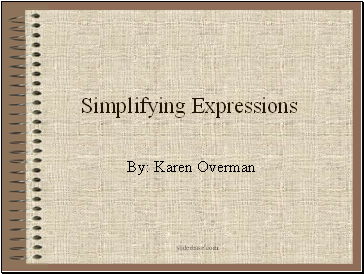Simplifying Expressions

By: Karen Overman

Slide 2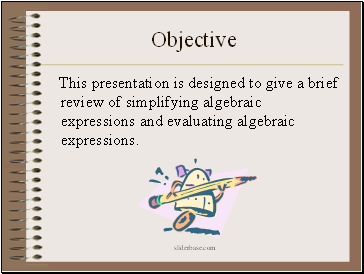Objective

This presentation is designed to give a brief review of simplifying algebraic expressions and evaluating algebraic expressions.

Slide 3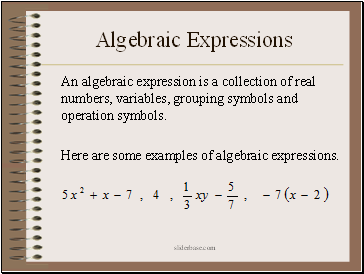## Algebraic Expressions

An algebraic expression is a collection of real numbers, variables, grouping symbols and operation symbols.

Here are some examples of algebraic expressions.

Slide 4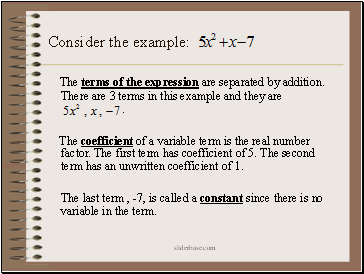Consider the example:

The terms of the expression are separated by addition. There are 3 terms in this example and they are .

The coefficient of a variable term is the real number factor. The first term has coefficient of 5. The second term has an unwritten coefficient of 1.

The last term , -7, is called a constant since there is no variable in the term.

Slide 5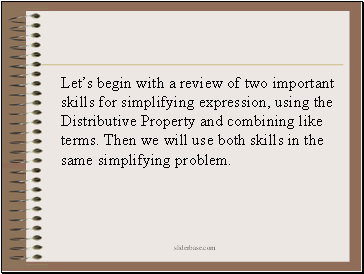Let’s begin with a review of two important skills for simplifying expression, using the Distributive Property and combining like terms. Then we will use both skills in the same simplifying problem.

Slide 6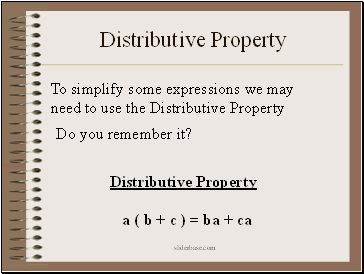## Distributive Property

a ( b + c ) = ba + ca

To simplify some expressions we may need to use the Distributive Property

Do you remember it?

Distributive Property

Slide 7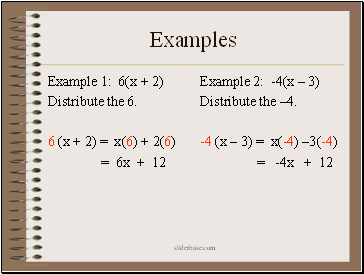Examples

Example 1: 6(x + 2)

Distribute the 6.

6 (x + 2) = x(6) + 2(6)

= 6x + 12

Example 2: -4(x – 3)

Distribute the –4.

-4 (x – 3) = x(-4) –3(-4)

= -4x + 12

Slide 8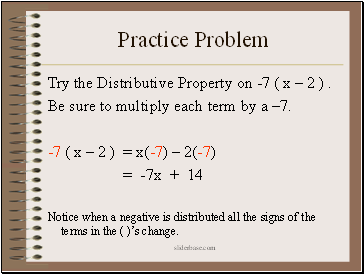## Practice Problem

Try the Distributive Property on -7 ( x – 2 ) .

Be sure to multiply each term by a –7.

-7 ( x – 2 ) = x(-7) – 2(-7)

= -7x + 14

Notice when a negative is distributed all the signs of the terms in the ( )’s change.

Slide 9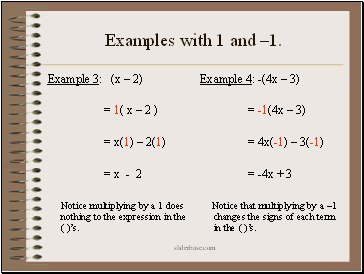Examples with 1 and –1.

Example 3: (x – 2)

= 1( x – 2 )

= x(1) – 2(1)

= x - 2

Notice multiplying by a 1 does nothing to the expression in the ( )’s.

Example 4: -(4x – 3)

= -1(4x – 3)

= 4x(-1) – 3(-1)

= -4x + 3

Notice that multiplying by a –1 changes the signs of each term in the ( )’s.

Slide 10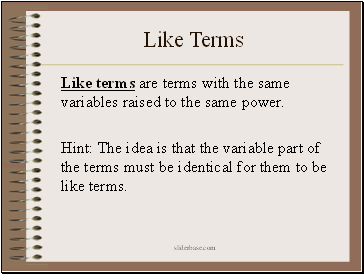## Like Terms

Like terms are terms with the same variables raised to the same power.

Go to page:
1  2  3